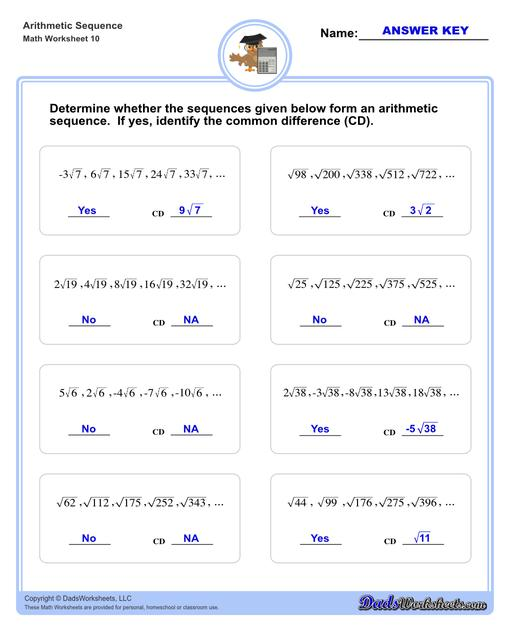PLEASE GO BACK AND USE THE BIG BLUE 'PRINT' BUTTON ON THE PAGE TO PRINT THE WORKSHEET CORRECTLY!Sorry for the trouble! The browser won't print the embedded worksheet PDF directly using the normal 'Print' command in the file menu, so you need to click the big 'Print' button to send just the worksheet and not the surrounding page to the printer.

# Math Worksheets: Arithmetic Sequences : Arithmetic Sequence Determine V2## Math Worksheets: Arithmetic Sequences: Arithmetic Sequence Determine V2

PropertyValue
DescriptionArithmetic sequences worksheets including practice finding the common difference for a sequence of numbers, or finding arbitrary nth terms in an arithmetic sequence given its formula definition. Arithmetic Sequence Determine V2
Resource TypeWorksheet